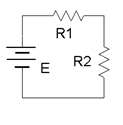# 8.4: Schematics and DiagramsFigure $$\PageIndex{1a}$$: (Four channel version shown)Figure $$\PageIndex{1b}$$Figure $$\PageIndex{2}$$